Courses

# CONCENTRATION FORMULAE Notes | EduRev

## Class 11 : CONCENTRATION FORMULAE Notes | EduRev

The document CONCENTRATION FORMULAE Notes | EduRev is a part of Class 11 category.
All you need of Class 11 at this link: Class 11

CONCENTRATION FORMULAE
There are several methods to express the concentration of solutions. We are going to describe some very important terms used to represent the concentration of solutions.
(a) Percent by weight
(b) Percent by volume
(c) Percent by weight/volume
(d) Mole fraction
(e) Molarity
(f) Molality
(g) Normality
(h) ppm

(a) Percent by Weight (w / w) : It is the weight of the solute as a per cent of the total weight of the solution. That is,
% By weight of solute =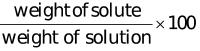For example, if a solution of HCI contains 36 % HCI by weight, it has 36 g of HCI in 100 g of solution.

(b) Percent by Volume (v / v) : It is the volume of solute present per 100ml of solution
% By volume =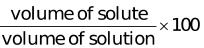For example, it we have 35% C2H5OH solution by volume means 35 ml C2H5OH is present per 100 ml of the solution.

(c) Percent by weight / volume (w / v) : It is the mass of solute present per 100g of solution, or % =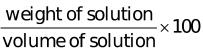i.e.30% HCl solution, means 30 g of HCl is present per 100 ml of solution.

(d) Mole Fraction (X) : A simple solution is made of two substances; one is the solute and the other is solvent. Mole fraction, X, of solute is defined as the ratio of the number of moles of solute and the total number of moles of solute and solvent. Thus,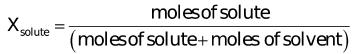If n represents moles of solute and N number of moles of solvent,
Then
Xsolute = n / n + N
Notice that mole fraction of solvent would be
Xsolvent = n / n + N
Mole fraction is unitless and (Xsolute + Xsolvent) = 1

(e) Molarity (M) : In current practice, concentration is most often expressed as molarity. Molarity is defined as the number of moles of solute per litre of solution.
Molarity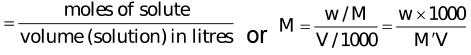w = Mass of solute in grams
M` = molecular weight of solute in gm/mol.

V = volume of solution in ml.
0.01 M NaOH, solution means that 0.01 mole NaOH is present in 1000 ml of its solution.

(f) Molality (m) : Molality of a solution is defined as the number of moles of solute per kilogram of solvent:
Molality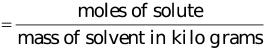or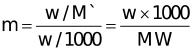where
w = mass of solute in grams
M` = molecular wt of solute in gm / mol
W = mass of solvent in grams.

(g) Normality (N) : Normality of a solution is defined as number of equivalents of solute per litre of the solution:
Normality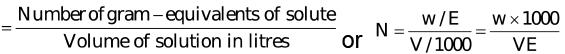w = mass of solute in gram
V = volume of solution in ml
E = equivalent wt of solute

(h) ppm (parts per million) : It is the mass of solute in grams present per 106 grams of solution.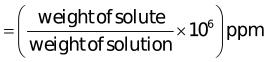and also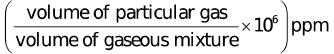⮚ Often in a chemistry laboratory, a solution of a desired concentration is prepared by diluting a solution of known higher concentration. The solution of higher concentration is also known as stock solution. Note that molality of a solution does not change with temperature since mass remains unaffected with temperature

Example.1. A solution is prepared by adding 2 g of a substance A to 18 g of water. Calculate the mass per cent of the solute.
Solution. Mass per cent
= 2 / -2 g of A +18 x 100
= 2g / 20g × 100

= 20g
= 10 %

Example.2. Calculate the molarity of NaOH in the solution prepared by dissolving its 4 g in enough water to form 250 mL of the solution.
Solution.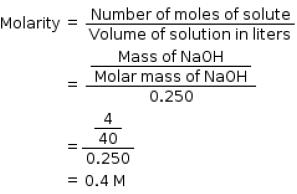Example.3. The density of 3 M solution of NaCl is 1.25 g mL–1. Calculate molality of the solution.
Solution.
M = 3 mol L–1

Mass of NaCl in 1 L solution = 3 × 58.5 = 175.5 g
Mass of 1L solution = Mass of solution × density of solution
= 1000 × 1.25 = 1250 g
(since density = 1.25 g mL–1)
Mass of water in solution = 1250 –175.5 = 1074.5 g
Molality = mass of solute in number of moles / mass of solvent in kg
= 3 / 1.07468 kg
= 2.7915 Molal.

Example.4. A solution of NaCl 0.5% by wt. If the density of the solution is 0.997 g/ml, calculate
(a) The molality
(b) Molarity
(c) Normality
(d) Mole fraction of the solute.

Solution.
Number of moles of NaCI = mass of NaCl / Molecular mass of Nacl
= 0.5 / 58.5 = 0.00854
(a) By definition,
Molality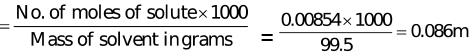Volume of the solution = Mass of solution in grams / Density in gm per m
= 100 / 0.997 = 100.3
(b) Now Molarity = Number of moles of solute / Volume of solution in litres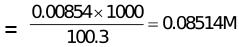(c) Normality = Number of gram equivalents of solute / volume of solution in litres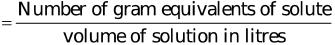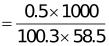= 0.0852

(d) To calculate mole fraction of the solute
No. of moles of water in 99.5g = 99.5 / 18 = 5.5277
Moles of NaCl = 0.5 / 58.5 = 8.547 x 10-3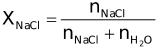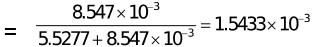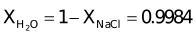Example.5. A sample of tooth-paste contains 10–4% F ions by wt. Find its ppm concentrations.
Solution.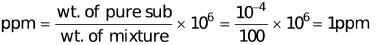Example.6. Calculate the molarity of a solution containing 0.5 g of NaOH dissolved in 500 cm3.
Solution. Weight of NaOH dissolved = 0.5 g Volume of the solution = 500 cm3 Calculation of molarity: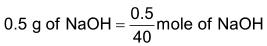(∵ Mol.mass of NaOH = 40)
= 0.0125 mole
Thus 500 cm3 of solution contain NaOH = 0.0125 mole
∴ 1000 cm3 of the solution contain NaOH
=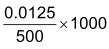⇒ 0.025 mole
Hence molarity of the solution = 0.025 M

Example.7. Calculate the molarity of a solution containing 9.8 gm of H2SO4 in 250 cm3 of the solution.
Solution. Mass of H2SO4 dissolved = 9.8 g
Volume of the solution = 250 cm3
Calculation of molarity
Mol. mass of H2SO= 98
∴ No. of moles of H2SO4 = Mass in g / Mol. mass
= 9.8 / 98 = 0.1
250 cm3 of the solution contain H2SO4
= 0.1 mole
∴ 1000 cm3 of the solution contain H2SO4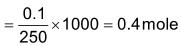Hence molarity of solution = 0.4 M

Example.8. Find the molarity and molality of a 15% solution of H2SO4 (density of H2SO4 = 1.020 g cm−3) (Atomic mass: H = 1, O = 16, S = 32 amu).
Solution. 15% of solution of H2SO4 means 15 g of H2SO4 are present in 100 g of the solution i.e.

Mass of H2SO4 dissolved = 15 g
Mass of the solution = 100 g
Density of the solution = 1.02 g/cm3 (given)
Calculation of molality:
Mass of solution = 100 g
Mass of H2SO4 = 15g
Mass of water (solvent) = 100 – 15 = 85 g
Mol. mass of H2SO= 98
∴ 15 g H2SO4 = 15 / 98 = 0.153 moles
Thus 85 g of the solvent contain 0.153 moles 100 g of the solvent contain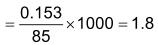Hence the molality of H2SO4 solution = 1.8 m
Calculation of molarity:
15 g of H2SO= 0.153 moles
Vol. of solution = Wt. of solution / Density of solution = 100 / 1.02 = 98.04 cm3

Thus 98.04 cm3 of solution contain H2SO4 = 0.153 moles.

Try Yourself

Q.1. The density of 3M solution of Na2S2O3 is 1.25g ml–1. Calculate
(a) The % by weights of NaSO3
(b) Mole fraction of NaSO3
(c) The molalities of Na+ and ions.
Ans.

(a) Mass of 1000 mL of Na2 S2 O3 solution = 1.25 × 1000 = 1250g
Mass of Na2 S2 O3 in 1000 mL of 3 M solution
= 3 × Mol mass of Na2 S2 O3
= 3 × 158 = 474g
Mass percentage of Na2 S2 O3 in solution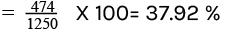(b) (ii) No. of moles of Na2 S2 O3 = 474 / 158 = 3
Mass of water =(1250−474)=776g
No. of moles of water = 776 / 18 = 43.1
Mole fraction of Na2 S2 O3 = 3 / 43.1 + 3 = 0.065

(c) No. of moles of Na+ ions
= 2 × No.of moles of Na2 S2 O3 = 2 × 3 = 6

Molality of Na+ ions =   No. of moles of Na+ ions / Mass of water in kg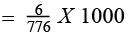= 7.73m
No. of moles of S2 O3 2− ions = No. of mole of Na2 S2 O3 = 3
Molality of S2 O3 2− -ions =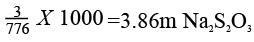Q.2. A sample of H2SO4 (density 1.787g ml–1) is labelled as 86% by weight. What is molarity of acid ? What volume of acid has to be used to make 1 litre of 0.2M H2SO4?
Ans.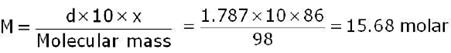Let V1 ml of this H2S04 are used to prepare 1L of 0.2 M
H2S04.M1V1 = M2V2
15.68 x V1 = 0.2 x 1000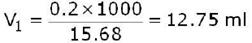Q.3. What weight of AgCl will be precipitated when a solution containing 4.77 g NaCl is added to a solution of 4.77 g of AgNO3 ?
Ans.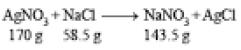From the given data, we find AgNO3 is limiting reagent as NaCl is in excess.
∴ 170.0 g of AgN03 precipitates AgCl = 143.5 g
∴ 5.77 g 0f AgN03 precipitates AgCl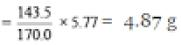Q.4. How much BaCl2 would be needed to make 250 ml of a solution having same concentration of Cl as the one containing 3.78 g of NaCl per 100 ml ?
Ans.
Moles of NaCl = 3.78 / 58.5 = 0.0646
1 NaCl contains one Cl
∴ moles of Cl  ions =0.0646
∴ (Cl) = no. of moles / Volume in litres = 0.064615 / 0.1

∴ concentration of Cl = [Cl] = 0.646
Again, BaCl ⇄ Ba + 2Cl
∴ [BaCl] = [Ba] = [Cl] / 2
From above concentration of Cl = 0.646 M
∴ [BaCl] = [Cl] / 2 = 0.646 / 2 = 0.323 M
Now, molarity of BaCl = weight of BaCl / molar weight of BaCl × volume in L 0.323 = weight of BaCl / (208.3 × 0.25)
[ ∵ molar weight of BaCl2 = 208.3g / mol and volume of solution = 250ml = 0.25L

∴0.323 × 208.3 × 0.25 = weight of BaCl2
weight of BaCl2 = 16.8g
Hence, weight of BaCl = 16.8g.

Offer running on EduRev: Apply code STAYHOME200 to get INR 200 off on our premium plan EduRev Infinity!

,

,

,

,

,

,

,

,

,

,

,

,

,

,

,

,

,

,

,

,

,

;